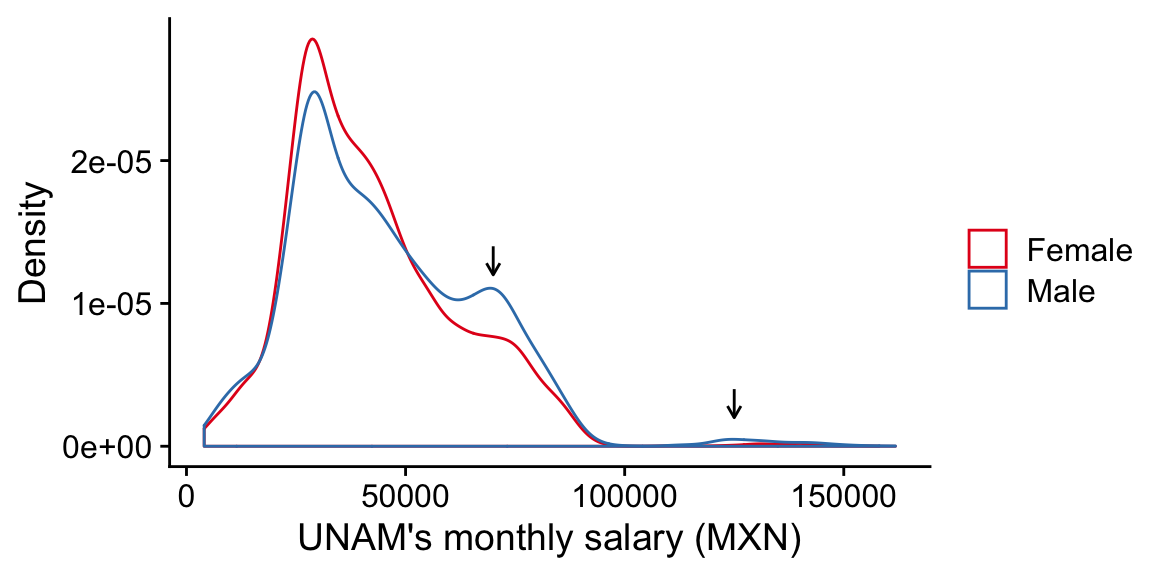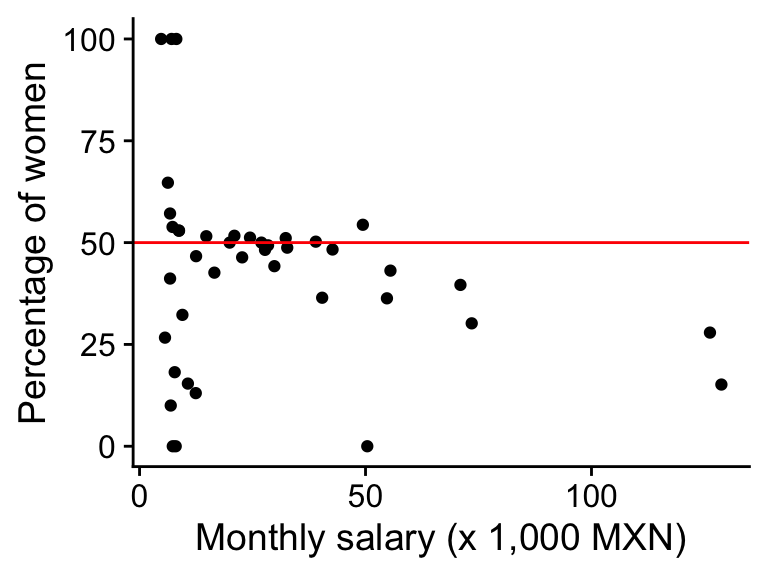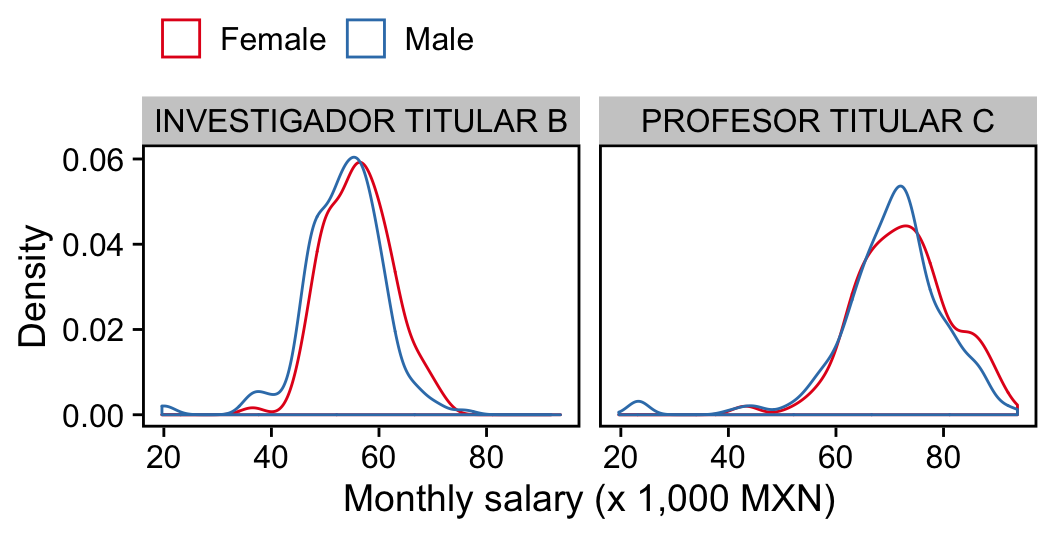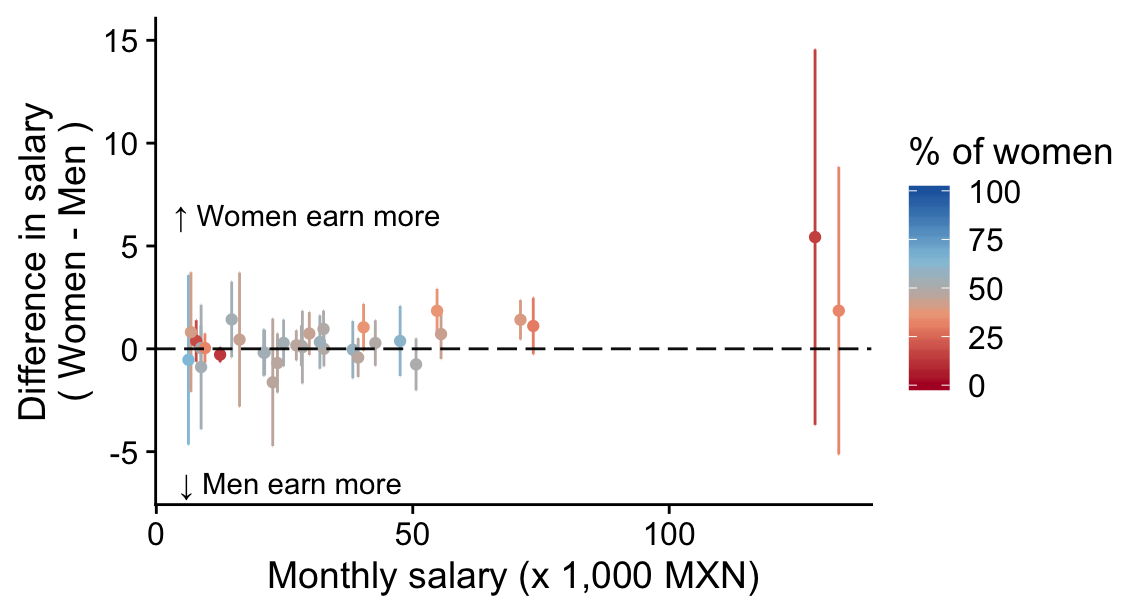# Unam’s Gender Pay Gap In 2019

Published:

UNAM’s faculty gender pay gap in the year 2019

The gender wage gap is the average difference in income between men and women. According to the Organisation for Economic Cooperation and Development, in 2018 the gender wage gap was 13.5% globally and 14% in Mexico 1. The reasons behind the wage gap include discrimination, occupational segregation and gender roles established by society. Research studies have estimated that gender wage gaps may not disappear before 2109 2.

The National Autonomous University of Mexico (UNAM, for its acronym in Spanish) is one of the biggest universities in Latin America. More than 10,000 researchers work in UNAM’s laboratories. Because UNAM is funded by taxpayers, the University is required to release all information about contracts and wages of each faculty member. These data are available in the UNAM’s transparency website.

The current blog post describes a documented data analysis that addresses the question: Is UNAM paying its male and female faculty equally? The code to reproduce this analysis can be found in my Github account.

# UNAM’s wages data

All UNAM’s wage data is publicly available. I downloaded the data corresponding to the year 2019 and saved them as tab-separated file. I did some additional data wrangling to remove non-ASCII characters and have nicer column names.

library(magrittr)

###perl -pi -e 's/[^[:ascii:]]//g' UNAM_Remuneracion-profesores_2019-09-11_21.41.55.txt
payData <- payData[,vapply( payData, function(x){length(unique(x))}, numeric(1)) > 1]
payData <- payData %>%
dplyr::rename(
unidad_academica=Unidad.acadmica,
nombre=Nombre.completo.del.profesor.a,
apellido_paterno=Primer.apellido.del.profesor.a,
apellido_materno=Segundo.apellido.del.profesor.a,
contrato=Tipo.o.nivel.de.contratacin,
pago_bruto=Remuneracin.bruta,
pago_neto=Remuneracin.neta,
estimulos=Estmulos.correspondientes.a.los.niveles.de.contratacin,
pago_total=Monto.total.percibido)

payData <- payData %>%
dplyr::mutate_at(
c("pago_bruto", "pago_neto", "pago_total"),
function(x){as.numeric(gsub("\\$|,", "", as.character(x)))}) payData$id <- sprintf("investigador%0.9d", seq_len(nrow(payData)))

payData$academic_title <- payData$contrato
payData$academic_title <- gsub( "^\\S+ (.*)$", "\\1", payData$academic_title ) These data contain information for 12,720 researchers, including full names, monthly salaries, academic ranks and academic departments. # Women constitute 45% of UNAM’s faculty Then, I aimed at assigning gender to each faculty member based on their first names. In my first attempt, I created catalogs of masculine and feminine names by scraping data from Wikipedia (the code for that can be found here). But I was pointed to an R package called gender, which uses US social security and census data to assign a gender to names. I used the gender package because it was better and cleaner than my name catalogs. In Mexico, it is common for people to have more than one first name and names like “María José” can be tricky, since “María” is a feminine name, “José” is a masculine name and the combination of the two, “María José”, is a name given to women. In these cases, I considered both the gender of the first first name and the consensus gender of the individual names. In general, these two strategies gave almost identical results. For researchers with a single first name, the assignment was straightforward. For researchers with more than one first name, I selected the gender of the first first name or the consensus gender of the names whenever the first first name was uninformative. Note that in my analysis, gender defaults to “man” and “woman” and as a result, I failed to include people who experience their gender identity as falling outside these two categories. namesDf <- as.data.frame(payData[,c("nombre", "id")]) namesDf$nombre <- strsplit( as.character(payData$nombre), " " ) namesDf <- tidyr::unnest(namesDf, cols = c(nombre)) namesDf <- namesDf %>% dplyr::mutate( nameOrder=ave(id, id, FUN=seq_along) ) library(gender) gendersPackage <- gender( unique(namesDf$nombre), 2012 )[,c("name", "gender")]

gendersPackage <- gendersPackage %>%
dplyr::mutate( gender=gsub("^female", "Female", gsub("^male", "Male", gender) ) ) %>%
dplyr::select( name, gender ) %>%
dplyr::rename( nombre=name )

namesDf <- dplyr::left_join( namesDf, gendersPackage )

genderFirstName <- namesDf %>%
dplyr::filter( nameOrder == 1 ) %>%
dplyr::select( id, gender )

genderConsensus <- namesDf %>%
dplyr::group_by( id, gender ) %>%
dplyr::summarize( cnt=dplyr::n() ) %>%
na.omit() %>%
reshape2::dcast( id ~ gender, value.var="cnt", fun.aggregate = length) %>%
dplyr::mutate(
genderConsensus=dplyr::case_when(
Male > Female ~ "Male",
Female > Male ~ "Female",
(Male == Female & Female > 0) ~ "Ambiguous",
is.numeric(Male) ~ "Unknown" )
)

genderAssignment <- dplyr::full_join( genderConsensus, genderFirstName )

genderAssignment$genderFinal <- with( genderAssignment, ifelse( genderConsensus %in% c("Ambiguous", "Other"), gender, genderConsensus ) ) payData <- payData %>% dplyr::left_join( genderAssignment[,c("id", "genderFinal")] ) %>% dplyr::rename( gender=genderFinal ) %>% dplyr::select( -pago_bruto ) payData$gender[is.na(payData$gender)] <- "Unknown" Using this approach, I could assign a gender to 12,320 faculty members, or 97% of UNAM’s academics. Of those, 5,498 (45%) were females. This estimate of the percentage of female faculty is consistent with UNAM’s reports. # High-rank positions are mostly occupied by men I found a significant difference between the wages earned by women and men ($$p = 2.9 * 10^{-13}$$). On average, male faculty earn 3,080 Mexican pesos (MXN) more than female faculty. The plot below shows the distribution of wages for each gender. library(cowplot) library(ggplot2) theme_set(theme_cowplot()) payData %>% dplyr::filter( gender %in% c("Male", "Female") ) %>% ggplot( aes( pago_total, col=gender ) ) + geom_density() + geom_segment( aes(x = x1, y = y1+.2e-5, xend = x1, yend = y1), data = data.frame(x1=c(70000, 125000), y1=c(1.2e-5, .2e-5) ), inherit.aes=FALSE, arrow = arrow(length = unit(0.03, "npc") ) ) + labs(y="Density", x="UNAM's monthly salary (MXN)", col="") + scale_colour_manual(values=c(Female="#e41a1c", Male="#377eb8"))These distributions show several modes, and there are two peaks towards the higher-ranking salaries that are higher for men than for women (see the arrows). These patterns suggest that higher-rank positions are occupied by men. Thus, I plotted the percentage of women in each academic title as a function of the average salary in that title. library(cowplot) theme_set(theme_cowplot()) contractSummary <- payData %>% dplyr::filter( gender %in% c("Male", "Female") ) %>% dplyr::group_by( academic_title ) %>% dplyr::summarise( num=dplyr::n(), avePay=mean( pago_total ) ) %>% dplyr::arrange( desc(avePay) ) payPerGenderSumm <- payData %>% dplyr::filter( gender %in% c("Male", "Female") ) %>% dplyr::group_by( academic_title, gender ) %>% dplyr::summarise( num=dplyr::n() ) %>% tidyr::pivot_wider(names_from="gender", values_from="num", values_fill=list(num=0)) %>% dplyr::mutate( femalePercent=100*(Female/(Male+Female) )) %>% dplyr::right_join( contractSummary ) %>% dplyr::ungroup() payPerGenderSumm %>% # dplyr::filter( num > 3 ) %>% ggplot( aes( avePay/1000, femalePercent) ) + geom_point() + geom_hline(yintercept=50, col="red") + ylim(0, 100) + labs(x="Monthly salary (x 1,000 MXN)", y="Percentage of women")In the plot above, the split between man and women is centered around 50% for most positions. But among the top 6 academic titles that are better paid, the percentage of women is way below 50%. The code below pulls the contracts where the average salary is above 50,000 MXN per month. For all contracts except one, the percentage of women is below 40%. The academic titles in this list are the most prestigious positions such as Emeritus Faculty and Investigadores/Profesores Titulares (equivalent to full professors in the US system). payPerGenderSumm %>% dplyr::filter( avePay > 50000) %>% dplyr::select( academic_title, num, femalePercent, avePay ) ## # A tibble: 7 x 4 ## academic_title num femalePercent avePay ## <chr> <int> <dbl> <dbl> ## 1 INVESTIGADOR EMERITO 66 15.2 128744. ## 2 PROFESOR EMERITO 43 27.9 126215. ## 3 INVESTIGADOR TITULAR C TIEMPO COMPLETO 792 30.2 73496. ## 4 PROFESOR TITULAR C TIEMPO COMPLETO 1671 39.6 71001. ## 5 PROFESOR TITULAR B TIEMPO COMPLETO 763 43.1 55514. ## 6 INVESTIGADOR TITULAR B TIEMPO COMPLETO 614 36.3 54739. ## 7 PROFESOR EXTRAORDINARIO 1 0 50389. Interestingly, several part-time senior positions are mostly occupied by men. payPerGenderSumm %>% dplyr::filter( femalePercent < 40, grepl("MEDIO TIEMPO", academic_title ) ) %>% dplyr::select( academic_title, femalePercent, avePay ) ## # A tibble: 8 x 3 ## academic_title femalePercent avePay ## <chr> <dbl> <dbl> ## 1 PROFESOR TITULAR C MEDIO TIEMPO 13.0 12438. ## 2 PROFESOR TITULAR B MEDIO TIEMPO 15.4 10695. ## 3 PROFESOR TITULAR A MEDIO TIEMPO 32.3 9482. ## 4 TECNICO ACADEMICO TITULAR A MEDIO TIEMPO 0 7995. ## 5 PROFESOR ASOCIADO B MEDIO TIEMPO 18.2 7779. ## 6 INVESTIGADOR ASOCIADO B MEDIO TIEMPO 0 7350. ## 7 PROFESOR ASOCIADO A MEDIO TIEMPO 10 6900. ## 8 TECNICO ACADEMICO AUXILIAR A MEDIO TIEMPO 26.7 5629. The analysis shows an overall difference of male faculty earning more money than female faculty. This difference is explained by male faculty members having higher academic titles than female faculty members. # Women earn 542 MXN more than men with the same contract Then I asked a sligthly different question: is there a gender pay gap for faculty members who have the same contract? To answer this question, I fitted a linear model $y_{i} = \beta_{0} + \beta_{1}^{female}x_{1i} + \sum_{j=2}^{q} {\beta_{j}^{contract}x_{ji}} + \epsilon_{i},$ where $$y_{i}$$ is the salary of individual $$i$$ and $$q$$ is the number of possible contracts. $$\beta_{0}$$ is the intercept term, which estimates the mean salary for men that have a contract that is arbitrarily selected as base level. $$x_{1i}$$ is a dummy variable that is equal to $$1$$ if individual $$i$$ is a female and is equal to $$0$$ if individual $$i$$ is a male. $$x_{ji}$$ are $$q-1$$ dummy variables that are equal to 1 if individual $$i$$ has a contract $$j$$ and are equal to $$0$$ if individual $$i$$ does not have contract $$j$$. $$\epsilon_{i}$$ is the error term that is normally distributed. The coefficient of interest in the model is $$\beta_{1}^{female}$$, which estimates the difference in salary that women receive compared to men after adjusting for salary differences between different contracts, which are estimated by the $${\beta}_{j}^{contract}$$ coefficients. testable <- as.character( payData %>% dplyr::filter( gender %in% c("Male", "Female") ) %>% dplyr::group_by( contrato ) %>% dplyr::summarise( num=dplyr::n() ) %>% dplyr::filter( num > 15 ) %>% dplyr::pull( contrato ) ) minN <- min( payData[payData$contrato %in% testable,] %>%
dplyr::group_by( gender, contrato ) %>%
dplyr::summarise( n=dplyr::n() ) %>%
dplyr::pull( n ) )

stopifnot( minN > 0 )

fit <- lm( pago_total ~ contrato + gender,
data={
dplyr::filter(payData, gender %in% c("Male", "Female"), contrato %in% testable ) %>%
dplyr::mutate( gender=factor(gender, levels=c("Male", "Female"))) } )

coefFemale <- coefficients(fit)[["genderFemale"]]
coefFemale
##  541.6868
pvalLm <- broom::tidy(anova(fit)) %>%
dplyr::filter( term == "gender" ) %>%
dplyr::pull(p.value)
pvalLm
##  0.0001195584

The $$p$$-value of an analysis of variance indicates that there is a significant difference in the salaries that women earn compare to men that are employed with the same contracts. The resulting $$\beta_{1}^{female}$$ coefficient indicates that women earn 542 MXN more than men. I found this result to be counterintuitive given that historically, due to discrimination, men typically earn more than women.

To find out the reason for these wage gaps, I analyzed the difference in pay in each contract. For each contract, I tested whether there were differences in salaries between male and female faculty members.

contractSummary <- payData %>%
dplyr::filter( gender %in% c("Male", "Female") ) %>%
dplyr::group_by( contrato ) %>%
dplyr::summarise( num=dplyr::n(), avePay=mean( pago_total ) ) %>%
dplyr::arrange( desc(avePay) )

payPerGenderSumm <- payData %>%
dplyr::filter( gender %in% c("Male", "Female") ) %>%
dplyr::group_by( contrato, gender ) %>%
dplyr::summarise( num=dplyr::n() ) %>%
tidyr::pivot_wider(names_from="gender", values_from="num", values_fill=list(num=0)) %>%
dplyr::mutate( femalePercent=100*(Female/(Male+Female) )) %>%
dplyr::right_join( contractSummary ) %>%
dplyr::ungroup()

differentPays <- payData %>%
dplyr::filter( gender %in% c("Male", "Female"), contrato %in% testable ) %>%
dplyr::mutate(gender=factor(gender, levels=c("Female", "Male"))) %>%
dplyr::group_by( contrato ) %>%
dplyr::group_map( ~ cbind(
broom::tidy( t.test( pago_total ~ gender, data=.x )),
contrato=unique( .x$contrato ), stringsAsFactors=FALSE), keep=TRUE ) %>% dplyr::bind_rows() %>% dplyr::select( estimate, estimate1, estimate2, conf.low, conf.high, contrato, p.value ) %>% dplyr::mutate( q.value = p.adjust( p.value, method="BH")) %>% dplyr::filter( q.value < 0.1 ) %>% dplyr::left_join( payPerGenderSumm[,c("contrato", "avePay")] ) %>% dplyr::mutate( aveDiff=(100*estimate1/estimate2) - 100 ) nrow(differentPays) ##  2 Two full-time contracts, Investigador Titular B and Profesor Titular C, were significant at a false discovery rate of 10%. The plot below shows the distributions of wages for these two contracts. library(cowplot) theme_set(theme_cowplot()) payData %>% dplyr::filter( contrato == dplyr::pull(differentPays, contrato), gender %in% c("Male", "Female") ) %>% dplyr::mutate( contrato = gsub(" TIEMPO COMPLETO|^\\S+ ", "", contrato) ) %>% ggplot( aes(pago_total/1000, col=gender ) ) + geom_density() + facet_wrap( ~contrato ) + theme(legend.pos="top", axis.line=element_blank()) + labs(x="Monthly salary (x 1,000 MXN)", y="Density", col="") + panel_border(colour="black", size=1) + scale_colour_manual(values=c(Female="#e41a1c", Male="#377eb8"))In these two positions, the average salary of women is 3.4% and 2% higher than that of men, respectively. The plot below shows the differences between the salaries of women compared to men ($$y$$-axis) for each contract (represented as a dot) plotted as a function of the average salary of the contract ($$x$$-axis). The points are color-coded according to the percentage of women in that contract. The vertical solid lines represent the 95% confidence intervals of the mean differences. Dots above the horizontal dotted line indicate that the average salary is higher for women than men, and dots below the horizontal dotted line indicate that the average salary of women is lower compared to that of men. library(cowplot) theme_set(theme_cowplot()) cols <- c( colorRampPalette( c("#b2182b", "#f4a582", "#bababa"), bias=0.5)(100), rev(colorRampPalette( c( "#2166ac", "#92c5de", "#bababa"), bias=0.5)(100) ) ) payData %>% dplyr::filter( gender %in% c("Male", "Female"), contrato %in% testable ) %>% dplyr::mutate(gender=factor(gender, levels=c("Female", "Male"))) %>% dplyr::group_by( contrato ) %>% dplyr::group_map( ~ cbind( broom::tidy(t.test( pago_total ~ gender, data=.x )), contrato=unique( .x$contrato ), stringsAsFactors=FALSE ), keep=TRUE ) %>%
dplyr::bind_rows() %>%
dplyr::select( estimate, conf.low, conf.high, contrato, p.value ) %>%
dplyr::mutate( q.value = p.adjust( p.value, method="BH")) %>%
dplyr::left_join( payPerGenderSumm ) %>%
ggplot( aes( avePay/1000, estimate/1000, col=femalePercent) ) +
geom_errorbar(aes(ymin=conf.low/1000, ymax=conf.high/1000), width=.1) +
geom_point() +
geom_hline(yintercept=0, col="black", alpha=0.95, linetype="longdash") +
annotate("text", x=27, y=6.5, label="   ↑ Women earn more", color = "black") +
annotate("text", x=27, y=-6.5, label=" ↓ Men earn more  ", color = "black") +
ylim(-6.5, 15) +
labs(x="Monthly salary (x 1,000 MXN)",
y="Difference in salary\n( Women - Men )",
col="% of women")For 70% of the contracts (23 out of 33), the average salary was higher for women than for men. This explains why the $$\beta_{1}^{female}$$ coefficient is significant in the linear model. However, the 95% confidence intervals overlap with the zero line for 30 of the contracts, which is why only two contracts, Investigador Titular B and Profesor Titular C, are significant in the contract-wise tests after multiple-testing correction.

# Conclusions

My analysis shows that among UNAM’s faculty members, men earn on average 7% more than women faculty. This wage gap is explained by the high-rank academic titles being occupied mostly by men. For example, only 15% of UNAM’s research faculty members with the highest academic titles, Investigadores Emeritos, are women.

When I tested for differences in wages between male and female faculty with the same contracts, I found that women earn on average 1% more than men with the same contract. This difference is more pronounced in two contracts, Investigador Titular B and Profesor Titular C, where women earn 3.4% and 2% more than men, respectively.

In the academic positions where the percentage of men is high, which tend to be senior positions, the few women in those positions earn more than men on average. This is very intriguing and I don’t have an explanation for it. One hypothesis is that men are promoted more often than women. In this scenario, female researchers would remain in lower-rank positions for longer and thus would accumulate more salary increases.

The biggest problem, however, is the lack of representation of women in senior faculty positions: in order to reach gender equality, UNAM should promote more women to the higher-rank academic titles.

# Session Information

sessionInfo()
## R version 3.6.1 (2019-07-05)
## Platform: x86_64-apple-darwin15.6.0 (64-bit)
## Running under: macOS Mojave 10.14
##
## Matrix products: default
## BLAS:   /Library/Frameworks/R.framework/Versions/3.6/Resources/lib/libRblas.0.dylib
## LAPACK: /Library/Frameworks/R.framework/Versions/3.6/Resources/lib/libRlapack.dylib
##
## locale:
##  en_US.UTF-8/en_US.UTF-8/en_US.UTF-8/C/en_US.UTF-8/en_US.UTF-8
##
## attached base packages:
##  stats     graphics  grDevices utils     datasets  methods   base
##
## other attached packages:
##  cowplot_1.0.0 ggpubr_0.2.3  ggplot2_3.2.1 gender_0.5.3  magrittr_1.5
##
## loaded via a namespace (and not attached):
##   Rcpp_1.0.3       pillar_1.4.2     compiler_3.6.1   plyr_1.8.4
##   tools_3.6.1      zeallot_0.1.0    digest_0.6.22    lattice_0.20-38
##   nlme_3.1-142     evaluate_0.14    lifecycle_0.1.0  tibble_2.1.3
##  gtable_0.3.0     pkgconfig_2.0.3  rlang_0.4.1      cli_1.1.0
##  yaml_2.2.0       xfun_0.11        genderdata_0.5.0 withr_2.1.2
##  dplyr_0.8.3      stringr_1.4.0    knitr_1.26       generics_0.0.2
##  vctrs_0.2.0      grid_3.6.1       tidyselect_0.2.5 glue_1.3.1
##  R6_2.4.1         fansi_0.4.0      rmarkdown_1.17   tidyr_1.0.0
##  purrr_0.3.3      reshape2_1.4.3   backports_1.1.5  scales_1.0.0
##  codetools_0.2-16 htmltools_0.4.0  assertthat_0.2.1 colorspace_1.4-1
##  ggsignif_0.6.0   labeling_0.3     utf8_1.1.4       stringi_1.4.3
##  lazyeval_0.2.2   munsell_0.5.0    broom_0.5.2      crayon_1.3.4